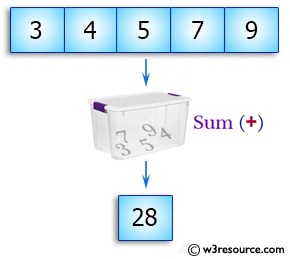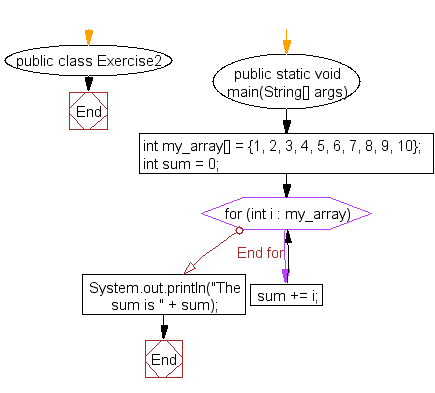﻿ Java exercises: Sum values of an array - w3resource# Java Array Exercises: Sum values of an array

## Java Array: Exercise-2 with Solution

Write a Java program to sum values of an array.

Pictorial Presentation:Sample Solution:

Java Code:

``````public class Exercise2 {
public static void main(String[] args) {
int my_array[] = {1, 2, 3, 4, 5, 6, 7, 8, 9, 10};
int sum = 0;

for (int i : my_array)
sum += i;
System.out.println("The sum is " + sum);
}
}
```
```

Sample Output:

```The sum is 55
```

Flowchart:Visualize Java code execution (Python Tutor):

Java Code Editor:

Improve this sample solution and post your code through Disqus

What is the difficulty level of this exercise?

﻿

## Java: Tips of the Day

Array vs ArrayLists:

The main difference between these two is that an Array is of fixed size so once you have created an Array you cannot change it but the ArrayList is not of fixed size. You can create instances of ArrayLists without specifying its size. So if you create such instances of an ArrayList without specifying its size Java will create an instance of an ArrayList of default size.

Once an ArrayList is full it re-sizes itself. In fact, an ArrayList is internally supported by an array. So when an ArrayList is resized it will slow down its performance a bit as the contents of the old Array must be copied to a new Array.

At the same time, it's compulsory to specify the size of an Array directly or indirectly while creating it. And also Arrays can store both primitives and objects while ArrayLists only can store objects.

Ref: https://bit.ly/3o8L2KH